### Home > CALC > Chapter 2 > Lesson 2.3.2 > Problem2-115

2-115.
1. While running in a straight line to class, Steven's distance from class (in meters) was recorded on the graph below. Homework Help ✎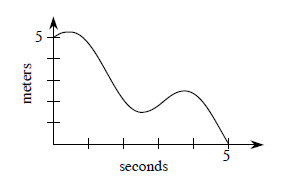1. Estimate his velocity (in meters per second) at t = 0, 1, and 3 seconds.

2. Did Steven ever stop and turn around? If so, when? How does the graph show this?

3. Approximate the interval(s) of time when Steven was headed toward class.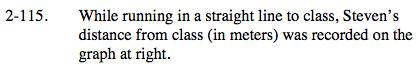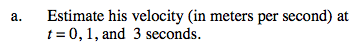Velocity is the steepness (or slope) of a distance graph. Since this graph is not linear, slope can only be approximated.

Think about ramp lab (2-112). Slope at a specific point was approximated by finding the slope of a line that connects two points close to the target.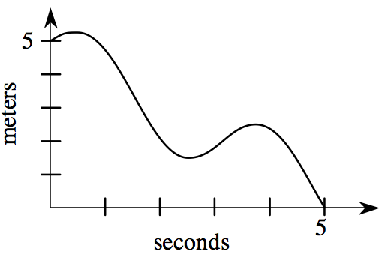We can approximate the slope at t = 1 by choosing two points close to t = 1 and finding the slope of the line that connects them.

$\text{velocity at } t = 1 \text{ is about } -\frac{5}{2} \text{ m/sec}$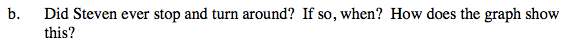This is a distance graph. y-values represent distance from the classroom.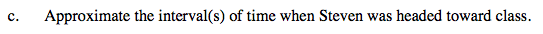The classroom is located at y = 0.